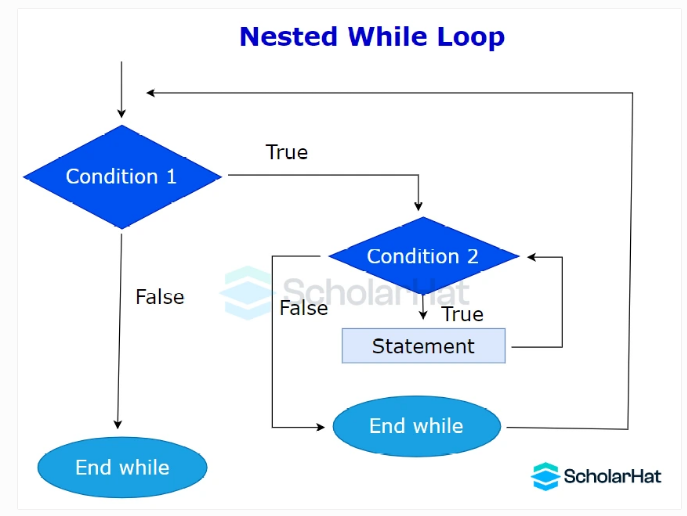# Understanding While Loop in C++

24 Nov 2023
Beginner
113 ViewsLearn via Video Course & by Doing Hands-on Labs

## While Loop in C++: An Overview

We have already become familiar with the concept of `loop` and `for loop`in C++ programming in the previous tutorial, Loops in C++. In this C++ tutorial, we are going to look at another important loop, `while loop` in C++. To strengthen your C++ programming skills, just consider our C++ Certification program and become a certified C++ programmer.

## While Loop in C++

It repeatedly carries out a series of instructions till a condition is `true`. It is an entry-controlled loop. The `while` loop in C++ is used when we don’t know the number of iterations.#### Syntax

``````while(testCondition){
//code to be executed
}
``````

If the `testCondition` inside the `()` becomes `true`, the body of the loop executes else loop terminates without execution. The process repeats until the `testCondition` becomes `false`.

#### Example to demonstrate while loop in C++

``` ```#include <iostream>
using namespace std;
int main() {
int i = 1;
while (i <= 10) {
cout << i << endl;
i++;
}
return 0;
}
``````
• In the above code, `i` is initialized to 1 before the start of the loop.
• The `testCondition`, `i<=10` is evaluated. If `true`, the body of the loop executes.
• If the condition becomes `false` in the beginning itself, the program control does not even enter the loop once.
• The loop executes until `i` becomes 10.

#### Output

``````1
2
3
4
5
6
7
8
9
10
``````

### Nested While Loop in C++

It is any type of loop inside a `while`loop.#### Example to demonstrate Nested do...while loop in C++

``` ```#include <iostream>
using namespace std;
int main() {
int i = 1, j = 1;
while (i <= 3) {
cout << "Outer loop iteration " << i << endl;
while (j <= 3) {
cout << " Inner loop iteration " << j << endl;
j++;
}
j = 1; // reset j to 1 for the next iteration of the outer loop
i++;
}
return 0;
}
``````
• The inner loop `j` iterates within each iteration of the outer loop `i`, printing "Inner loop iteration" messages
• The outer loop `i` iterates three times, printing "Outer loop iteration" messages.
• After each inner loop is finished, `j` is reset to 1, resulting in `nested` iteration.

#### Output

``````Outer loop iteration 1
Inner loop iteration 1
Inner loop iteration 2
Inner loop iteration 3
Outer loop iteration 2
Inner loop iteration 1
Inner loop iteration 2
Inner loop iteration 3
Outer loop iteration 3
Inner loop iteration 1
Inner loop iteration 2
Inner loop iteration 3
``````

### Infinite while loop in C++

If the condition of a loop is always `true`, the loop becomes an `infinite` one.

#### Syntax

``````while(true)
{
// body of the loop..
}
``````

#### Example to demonstrate infinite do...while loop in C++

``````#include <iostream>
using namespace std;
int main() {
char str[] = "Infinite while loop";
int i = 0;

while (true) {
i++;
cout << "i is: " << i << endl;
}

// The return statement may never be reached in an infinite loop.
return 0;
}
``````

Here, the value of `i` will be printed `n` number of times due to the absence of a terminating condition.

#### Output

``````i is: 1
i is: 2
i is: 3
i is: 4
i is: 5
...
``````
##### Summary
So, in this article, we have analyzed the `while` loop from all angles and have understood its applications in various scenarios. If you want to further understand the loop and its application in various C++ programs, you can consider our C++ Certification program.
Share Article
Similar Articles